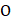# Following question is based on letter series.  In each question some letters are missing shown by ( – ).  The missing letter are given in a proper sequence as one of the four alternative given under each question.  Find out the correct alternative. K(a) B K C C (b) C B K C (c) B C K B (d) B C K C

## Question ID - 100726 :- Following question is based on letter series.  In each question some letters are missing shown by ( – ).  The missing letter are given in a proper sequence as one of the four alternative given under each question.  Find out the correct alternative. K(a) B K C C (b) C B K C (c) B C K B (d) B C K C

3537

B K C C

Next Question :

A disc of massand radiusis rolling with angular speedon a horizontal plane as shown Figure. The magnitude of angular momentum of the disc about the originisa)b)c)d)